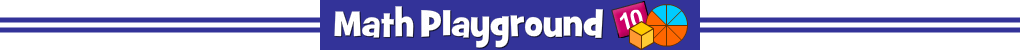Make groups of equal value.
Each group must contain a fraction, a decimal and a percent.
Triplets - Learning Connections
Essential Skills
Problem Solving - complete mathematical puzzles

Common Core Connection for Grades 3 and 4
Recognize and generate simple equivalent fractions.
Explain why the fractions are equivalent by using a visual fraction model.
Decompose a fraction into a sum of fractions with the same denominator.
Compare two fractions with different numerators and different denominators.
Recognize that comparisons are valid only when fractions refer to the same whole.

More Math Games to Play